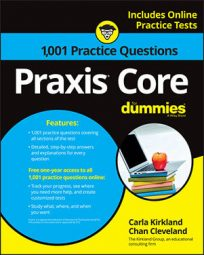##### Praxis Core: 1,001 Practice Questions For DummiesYou can think of a cylinder as a circle with attitude. If you encounter a cylinder problem on the Praxis Core exam, you can knock it down to size if you remember a few simple formulas.

In the first practice question, you apply the surface area formula to a right cylinder. In the second question, you need to use the volume formula—and a little subtraction—to get the right answer.

## Practice questions

Refer to the following figure for the first question.

1. What is the surface area of the right cylinder?

Refer to the following figure for the next question.

2. In the diagram, a cylinder is on the interior of another cylinder. The bases of the interior cylinder are parts of the bases of the larger cylinder. The centers of the bases are shared by the cylinders. How much volume of the larger cylinder is outside of the smaller cylinder?

1. The correct answer is Choice (A). The radius of a cylinder, as with just a circle, is half its diameter. The radius for the cylinder here is half of 8 km, so it's 4 km. Use the surface area formula for a right prism, where SA is the surface area, P is the perimeter of the base (the circumference in this case), h is the height, and B is the base area:

The surface area of the cylinder is 152 π km2.

2. The correct answer is Choice (C). The amount of volume of the larger cylinder that is outside of the smaller cylinder is the larger cylinder's volume minus the smaller cylinder's volume. To find that difference, first find the larger cylinder's volume:

The volume of the larger cylinder is

Next, find the volume of the smaller cylinder:

The volume of the larger cylinder is

The difference between the larger cylinder's volume and the smaller cylinder's volume is